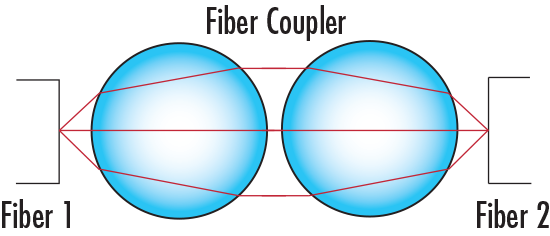# 2 Ball Lens Fiber Coupler Calculator

View All Technical Tools

Fiber 1 Numerical Aperture, NA1:
Fiber 2 Numerical Aperture, NA2:
Ball Lens 1 Material:
Ball Lens 1 Index of Refraction:

### --

Ball Lens 1 Diameter, D1:
Ball Lens 2 Material:
Ball Lens 2 Index of Refraction:

### --

Ball Lens 2 Diameter D2:

d: --

EFL1 (mm): --

BFL1 (mm): --

EFL2 (mm): --

BFL2 (mm): --

Ball Lens Output NA: --## Equations and Corresponding Legend

 $$\text{NA} = \frac{2 \, d \left(n - 1 \right)}{n \, D}$$
 $$d = \frac{n \, D}{4 \left(n - 1 \right) \sqrt{ \frac{ \left( \frac{1}{\text{NA}} \right)^2 - 1 }{4} } }$$
 $$\text{EFL} = \frac{n \, D}{4 \left( n - 1 \right)}$$
 $$\text{BFL} = \text{EFL} - \frac{D}{2}$$
 NA1 Represents numerical aperture of the first fiber NA2 Represents numerical aperture of the second fiber EFL1 Represents the effective focal length of the first ball lens EFL2 Represents the effective focal length of the second ball lens BFL1 Represents the back focal length of the first ball lens BFL2 Represents the back focal length of the second ball lens
 n1 Represents the refractive index of the first ball lens n2 Represents the refractive index of the second ball lens D1 Represents the diameter of the first ball lens D2 Represents the diameter of the second ball lens d Diameter of the beam between the ball lenses

## Description

Identify a compatible pair of ball lenses for coupling light from one optical fiber into another using the numerical aperture of each fiber, the ball lens material, and the ball lens diameter. Identical ball lenses are used when coupling light from one fiber optic to another fiber optic of similar NA, but different ball lenses may be needed when coupling light between fibers of different NA. The ball lens output NA must be less than or equal to the NA of the second fiber in order to maximize throughput.

## Related Resources and Products